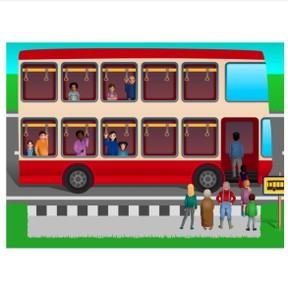On the bus: Addition to 20 making ten

# On the bus: Addition to 20 making ten8,000 schools use Gynzy92,000 teachers use Gynzy1,600,000 students use Gynzy

## General

Students can add to 20 by using the context of a bus ride.

## Relevance

If students want to be able to add well, it is important to be able to add to 20 using the context of a bus ride. The bus helps to make addition problems understandable and to visualize the addition.

## Introduction

Show an image of a double decker bus where people are getting on. Ask if anyone has been on a busy bus. Then practice composing 10 in different ways with students. Ask the class how many more students you need to compose 10.

## Development

Using the three images of busses on the interactive whiteboard, explain to students how the bus method works. You first count the passengers on the arriving bus, then you count the passengers waiting for the bus, and then you know how that the total number of passengers leaving with the bus is the first two numbers combined. The first addend of the problem is the number of passengers in the bus as it arrives to a stop. The second addend is the amount of passengers waiting for the bus at the stop. The total, or sum, is the combination of the two addends. Show this with an example where you first count to 10 and then continue counting on. Discuss two more bus problems where you can count the individuals from the image presented.
Tell the students that the image will now stay the same, that you can show a bus problem in one picture, and practice this with two bus problems. Next show students a bus problem in which they cannot count the passengers, but are given an abstract number. Explain that the first number is the first addend, the number of passengers on the bus, the second number is the second addend, the number of passengers waiting to get on. With this example, be sure to emphasize that students can add to 10, and then count on to make their addition easier. Practice the bus problems with abstract numbers as a class.

Check that students understand addition to 20 with bus problems by asking the following questions:
- Which steps do you take to solve a bus problem?
- Is the number of passengers on the bus the first or second addend?
- What do you do with the passengers that get on the bus?

## Guided practice

Students first determine which image matches the addition problem by selecting from multiple choice answers. Then they must determine the addition problem represented by an image on their own. Finally students must also calculate the sum of the bus problem.

## Closing

Repeat the learning goal and ask which steps you take to solve a bus problem. Ask, using two examples, how many passengers are on a bus after more passengers get on. Finally ask students to form small groups and to create bus problems using 10-sided dice. They throw twice.The first throw is the number of passengers, and the second is the amount of waiting passengers. They then solve the problem.

## Teaching tips

Students who have difficulty can be helped by repeating the steps of solving a bus problem. First counting the passengers on the bus, then counting how many are waiting for the bus, and finally counting all the passengers together. They can also be helped by the use of manipulatives to represent each passenger on the bus and waiting for the bus.

## Instruction materials

ten-sided dice, blocks

### The online teaching platform for interactive whiteboards and displays in schools

• Save time building lessons

• Manage the classroom more efficiently

• Increase student engagement